vector_vectory集合 - CSDNvector 订阅
Vector类 是在 java 中可以实现自动增长的对象数组，vector在C++标准模板库中的部分内容，它是一个多功能的，能够操作多种数据结构和算法的模板类和函数库。 展开全文Vector类 是在 java 中可以实现自动增长的对象数组，vector在C++标准模板库中的部分内容，它是一个多功能的，能够操作多种数据结构和算法的模板类和函数库。

JAVA、C++

vector

#include

using namespace std
vector详细介绍
vector是C++标准模板库中的部分内容，中文偶尔译作“容器”，但并不准确。它是一个多功能的，能够操作多种数据结构和算法的模板类和函数库。vector之所以被认为是一个容器，是因为它能够像容器一样存放各种类型的对象，简单地说，vector是一个能够存放任意类型的动态数组，能够增加和压缩数据。   简单的使用方法如下：我们可以用一个迭代器：vector::iterator iter=test.begin();//定义一个可以迭代int型vector的迭代器iter，它指向test的首位for(;iter!=test.end();iter++) cout<<(*iter);//iter++指的是向后迭代一位，直到iter到超出末端迭代器为止，输出迭代器指向的值我们也可以使用at访问：Java中Vector类是允许不同类型元素共存的变长数组，Java.util.Vector提供了向量（Vector）类以实现类似动态数组的功能。在Java语言中是没有指针概念的，但如果能正确灵活地使用指针又确实可以大大提高程序的质量，比如在C、C++中所谓“动态数组”一般都由指针来实现。为了弥补这点缺陷，Java提供了丰富的类库来方便编程者使用，Vector类便是其中之一。事实上，灵活使用数组也可完成向量类的功能，向量类中提供的大量方法也大大方便了用户的使用。   在相对于ArrayList来说，Vector线程是安全的，也就是说是同步的。创建了一个向量类的对象后，可以往其中随意地插入不同的类的对象，既不需顾及类型也不需预先选定向量的容量，并可方便地进行查找。对于预先不知或不愿预先定义数组大小，并需频繁进行查找、插入和删除工作的情况，可以考虑使用向量类。向量类提供了三种构造方法：   使用第一种方法，系统会自动对向量对象进行管理。若使用后两种方法，则系统将根据参数initialcapacity设定向量对象的容量（即向量对象可存储数据的大小），当真正存放的数据个数超过容量时，系统会扩充向量对象的存储容量。参数capacityIncrement给定了每次扩充的扩充值。当capacityIncrement为0时，则每次扩充一倍。利用这个功能可以优化存储。在Vector类中提供了各种方法方便用户使用：

• vector 是向量类型 它可以容纳许多类型的数据，如若干个整数，所以称其为容器。vector 是C++ STL的一个重要成员，使用它时需要包含头文件：#include; 一、vector 的初始化： (1) vector<int> a(10); //定义...
vector 是向量类型
它可以容纳许多类型的数据，如若干个整数，所以称其为容器。vector 是C++ STL的一个重要成员，使用它时需要包含头文件：#include;
一、vector 的初始化：
(1)  vector<int> a(10);
//定义了10个整型元素的向量（尖括号中为元素类型名，它可以是任何合法的数据类型），但没有给出初值，其值是不确定的。
（2 )  vector<int> a(10,1);
//定义了10个整型元素的向量,且给出每个元素的初值为1
（3）vector<int> a(b); //用b向量来创建a向量，整体复制性赋值
（4）vector<int> a(b.begin(),b.begin+3);
//定义了a值为b中第0个到第2个（共3个）元素
（5）int b={1,2,3,4,5,9,8}; vector<int> a(b,b+7); //从数组中获得初值

二、vector对象的几个重要操作：
（1）a.assign(b.begin(), b.begin()+3);
//b为向量，将b的0~2个元素构成的向量赋给a
（2）a.assign(4,2); //是a只含4个元素，且每个元素为2
（3）a.back(); //返回a的最后一个元素
（4）a.front(); //返回a的第一个元素
（5）a[i]; //返回a的第i个元素，当且仅当a[i]存在2013-12-07
（6）a.clear(); //清空a中的元素
（7）a.empty(); //判断a是否为空，空则返回ture,不空则返回false
（8）a.pop_back(); //删除a向量的最后一个元素
（9）a.erase(a.begin()+1,a.begin()+3); //删除a中第1个（从第0个算起）到第2个元素，也就是说删除的元素从a.begin()+1算起（包括它）一直到a.begin()+         3（不包括它）
（10）a.push_back(5); //在a的最后一个向量后插入一个元素，其值为5
（11）a.insert(a.begin()+1,5); //在a的第1个元素（从第0个算起）的位置插入数值5，如a为1,2,3,4，插入元素后为1,5,2,3,4
（12）a.insert(a.begin()+1,3,5); //在a的第1个元素（从第0个算起）的位置插入3个数，其值都为5
（13）a.insert(a.begin()+1,b+3,b+6); //b为数组，在a的第1个元素（从第0个算起）的位置插入b的第3个元素到第5个元素（不包括b+6），如b为1,2,3,4,5,9,8         ，插入元素后为1,4,5,9,2,3,4,5,9,8
（14）a.size(); //返回a中元素的个数；
（15）a.capacity(); //返回a在内存中总共可以容纳的元素个数
（16）a.resize(10); //将a的现有元素个数调至10个，多则删，少则补，其值随机
（17）a.resize(10,2); //将a的现有元素个数调至10个，多则删，少则补，其值为2
（18）a.reserve(100); //将a的容量（capacity）扩充至100，也就是说现在测试a.capacity();的时候返回值是100.这种操作只有在需要给a添加大量数据的时候才         显得有意义，因为这将避免内存多次容量扩充操作（当a的容量不足时电脑会自动扩容，当然这必然降低性能）
（19）a.swap(b); //b为向量，将a中的元素和b中的元素进行整体性交换
（20）a==b; //b为向量，向量的比较操作还有!=,>=,<=,>,<

三、顺序访问vector的几种方式：
（1）向向量a中添加元素
1、简单
 vector<int> a;
for(int i=0;i<10;i++)
a.push_back(i);

2、从数组中选择元素向向量中添加
int a={1,2,3,4,5,6};
vector<int> b；
for(int i=1;i<=4;i++)
b.push_back(a[i]);

3、从现有向量中选择元素向向量中添加
int a={1,2,3,4,5,6};
vector<int> b;
vector<int> c(a,a+4);
for(vector<int>::iterator it=c.begin();it<c.end();it++)
b.push_back(*it);

4、从文件中读取元素向向量中添加
ifstream in("data.txt");
vector<int> a;
for(int i; in>>i)
a.push_back(i);

5、【误区】
vector<int> a;
for(int i=0;i<10;i++)
a[i]=i;

//这种做法以及类似的做法都是错误的。刚开始我也犯过这种错误，后来发现，下标只能用于获取已存在的元素，而现在的a[i]还是空的对象。
（2）从向量中读取元素
1、通过下标方式读取
int a={1,2,3,4,5,6};
vector<int> b(a,a+4);
for(int i=0;i<=b.size()-1;i++)
cout<<b[i]<<" ";

2、通过遍历器方式读取
int a={1,2,3,4,5,6};
vector<int> b(a,a+4);
for(vector<int>::iterator it=b.begin();it!=b.end();it++)
cout<<*it<<" ";

四、几种重要的算法，使用时需要包含头文件：
  #include<algorithm>
（1）sort(a.begin(),a.end()); //对a中的从a.begin()（包括它）到a.end()（不包括它）的元素进行从小到大排列
（2）reverse(a.begin(),a.end()); //对a中的从a.begin()（包括它）到a.end()（不包括它）的元素倒置，但不排列，如a中元素为1,3,2,4,倒置后为4,2,3,1
（3）copy(a.begin(),a.end(),b.begin()+1); //把a中的从a.begin()（包括它）到a.end()（不包括它）的元素复制到b中，从b.begin()+1的位置（包括它）开        始复制，覆盖掉原有元素
（4）find(a.begin(),a.end(),10); //在a中的从a.begin()（包括它）到a.end()（不包括它）的元素中查找10，若存在返回其在向量中的位置



展开全文• ## vector

2020-05-23 18:01:13
1.vector概述 vector的数据安排以及操作方式与array非常类似。两者唯一的差别就是在于空间的运用灵活性，array是静态空间，一旦分配了就是固定的，无法改变其大小。需要用户重新申请更大的空间，移动数据，释放原来...
本文大部分内容转载于：https://blog.csdn.net/qq_41453285/article/details/103565158，他的具体内容也是把stl源码剖析进行了重点的摘要。
1.vector概述
vector的数据安排以及操作方式与array非常类似。两者唯一的差别就是在于空间的运用灵活性，array是静态空间，一旦分配了就是固定的，无法改变其大小。需要用户重新申请更大的空间，移动数据，释放原来的空间。而vector是动态空间，有其内部的空间分配机制来及时调整空间大小，其实就是vector把申请空间，移动数据，释放原来的空间等操作封装在内部，不需要用户去处理。
//alloc是SGI STL的空间配置器
template <class T, class Alloc = alloc>
class vector {
public:
// vector 的嵌套类型定义
typedef T value_type;
typedef value_type* pointer;
typedef value_type* iterator;
typedef value_type& reference;
typedef size_t size_type;
typedef ptrdiff_t difference_type;

protected:
// simple_alloc是SGI STL的空间配置器，见前面空间适配器文章的介绍
typedef simple_alloc<value_type, Alloc> data_allocator;
iterator start; // 表示目前使用空间的头
iterator finish; // 表示目前使用空间的尾
iterator end_of_storage; // 表示目前可用空间的尾

void insert_aux(iterator position, const T& x);
void deallocate() {
if (start)
data_allocator::deallocate(start, end_of_storage - start);
}

void fill_initialize(size_type n, const T& value) {
start = allocate_and_fill(n, value);
finish = start + n;
end_of_storage = finish;
}

public:
iterator begin() { return start; }
iterator end() { return finish; }
size_type size() const { return size_type(end() - begin()); }
size_type capacity() const {
return size_type(end_of_storage - begin());
}
bool empty() const { return begin() == end(); }
reference operator[](size_type n) { return *(begin() + n); }

vector() : start(0), finish(0), end_of_storage(0) {}
vector(size_type n, const T& value) { fill_initialize(n,value); }
vector(int n, const T& value) { fill_initialize(n,value); }
vector(long n, const T&value) { fill_initialize(n,value); }
explicit vector(size_type n) { fill_initialize(n,T()); }

~vector()
destroy(start, finish); //全局函式，见前面文章destroy函数的介绍
deallocate(); //这是 vector的㆒个 member function
}

reference front() { return *begin(); } // 第一个元素
reference back() { return *(end() - 1); } // 最后一个元素
void push_back(const T& x) { // 将元素安插至最尾端
if (finish != end_of_storage) {
construct(finish, x); //全局函式，见前面文章construct函数的介绍
++finish;
}
else
insert_aux(end(), x); //这是 vector的一个member function
}

void pop_back() { // 将最尾端元素取出
--finish;
destroy(finish); // 全局函式，见前面文章destroy函数的介绍
}

iterator erase(iterator position) { // 清除某位置上的元素
if (position + 1 != end())
copy(position + 1, finish, position); // 后续元素往前搬移
--finish;
destroy(finish); // 全局函式，见前面文章destroy函数的介绍
return position;
}

void resize(size_type new_size, const T& x) {
if (new_size < size())
erase(begin() + new_size, end());
else
insert(end(), new_size - size(), x);
}
void resize(size_type new_size) { resize(new_size, T()); }
void clear() { erase(begin(), end()); }

protected:
// 配置空间并填满内容
iterator allocate_and_fill(size_type n, const T& x) {
iterator result = data_allocator::allocate(n);
uninitialized_fill_n(result, n, x); // 全局函式，见前面uninitialized_fill_n函数的介绍
return result;
}

vector的迭代器
	vector内部只有四个成员变量，三个迭代器和1个空间配置器。
vector维护的是一个连续的线性空间，所以不论其元素类型是什么，普通指针都可以作为vector的迭代器并且满足迭代器的所有必要条件。
实际上，vector的迭代器就是原生指针，只不过换了个名字。在x86系统下，sizeof(vector<int>) 的结果为32。

    // vector 的嵌套类型定义
typedef T value_type;   //类型
typedef value_type* pointer;  //指针
typedef value_type* iterator; //迭代器

	vector存储数据的空间是线性连续的，因此支持随机的下标访问。

    typedef simple_alloc<value_type, Alloc> data_allocator;
iterator start; // 表示目前使用空间的头
iterator finish; // 表示目前使用空间的尾
iterator end_of_storage; // 表示目前可用空间的尾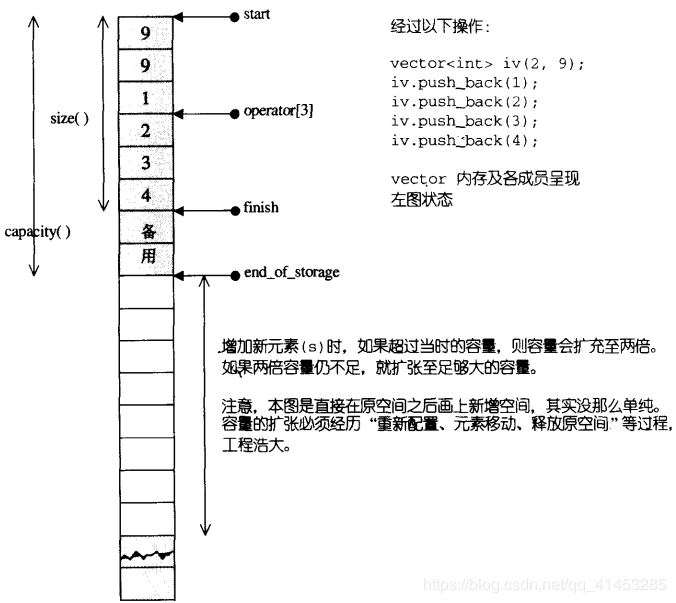运用start、finish、end_of_storage三个迭代器，vector提供了首尾标示、大小、容量、空容器判断、注标[]运算符、最前端元素值、最后端元素值…等机能，如下
template <class T, class Alloc = alloc>
class vector {
...
public:
iterator begin() { return start; }
iterator end() { return finish; }
size_type size() const { return size_type(end() - begin()); }
size_type capacity() const {
return size_type(end_of_storage - begin());
}
bool empty() const { return begin() == end(); }
reference operator[](size_type n) { return *(begin() + n); }
reference front() { return *begin(); }
reference back() { return *(end() - 1); }
...
};

vector的构造与内存管理
vector默认使用alloc做为空间配置器，并据此另外定义了一个data_allocator，为的是更方便以元素大小为配置单位：
template <class T, class Alloc = alloc>
class vector {
protected:
// simple_alloc<>见前面文章介绍
typedef simple_alloc<value_type, Alloc> data_allocator;
...
};

于是，data_allocator::allocate(n) 表示配置n个元素空间
vector的内存动态增长：当插入新元素时，空间已经不够用的时候，会申请一个新的空间，新空间的大小为当前空间大小的1.5倍（有些地方设置的是2倍），然后将原来的元素逐个拷贝到新空间里，释放原来的空间，插入新元素。
即 1、2、3、4、6、9…依次增长。在删除元素的时候并不会动态的调整空间的大小
vector内部空间变化：由于vector维护的空间是线性的连续的，因此也就引入一个新的问题，当在某个地方插入或删除新的元素的时候，会导致后面的元素需要逐个后移或前移，让出足够空间来插入这个新元素或者后面的元素前移来覆盖掉要删除的元素。
这些内部的空间变化都会导致相应的迭代器失效，即原先迭代器指向的数据发生了变化，需要注意。
vector的构造函数：
    vector() : start(0), finish(0), end_of_storage(0) {}
vector(size_type n, const T& value) { fill_initialize(n,value); }
vector(int n, const T& value) { fill_initialize(n,value); }
vector(long n, const T&value) { fill_initialize(n,value); }
explicit vector(size_type n) { fill_initialize(n,T()); }

常用的操作：
	//数据量
size_type size() const { return size_type(end() - begin()); }
//容量
size_type capacity() const {
return size_type(end_of_storage - begin());
}
//是否为空
bool empty() const { return begin() == end(); }
//[]下标访问
reference operator[](size_type n) { return *(begin() + n); }
// 第一个元素
reference front() { return *begin(); }
// 最后一个元素
reference back() { return *(end() - 1); }
// 将元素安插至最尾端
void push_back(const T& x) {
if (finish != end_of_storage) {
construct(finish, x); //全局函式，见前面文章construct函数的介绍
++finish;
}
else
insert_aux(end(), x); //这是 vector的一个member function
}
// 将最尾端元素取出
void pop_back() {
--finish;
destroy(finish); // 全局函式，见前面文章destroy函数的介绍
}
// 清除某位置上的元素
iterator erase(iterator position) {
if (position + 1 != end())
copy(position + 1, finish, position); // 后续元素往前搬移
--finish;
destroy(finish); // 全局函式，见前面文章destroy函数的介绍
return position;
}
//重新分配vector大小为n个指定的值
void resize(size_type new_size, const T& x) {
if (new_size < size())
erase(begin() + new_size, end());
else
insert(end(), new_size - size(), x);
}
//重新分配vector大小为n个默认的值
void resize(size_type new_size) { resize(new_size, T()); }
//清空vector
void clear() { erase(begin(), end()); }

insert函数的源代码
//从position开始，插入n个元素，元素初值为x
template<class T,class Alloc>
void vector<T, Alloc>::insert(iterator position, size_type n, const T& x)
{
if (n != 0) { //当n!= 0才进行以下所有动作
if (size_type(end_of_storage - finish) >= n){
//备用空间大于等于“新增元素个数”
T x_copy = x;
// 以下计算插入点之后的现有元素个数
const size_type elems_after = finish - position;
iterator old_finish = finish;
if (elems_after > n){
//“插入点之后的现有元素个数”大于“新增元素个数”
uninitialized_copy(finish - n, finish, finish);
finish += n; // 将vector尾端标记后移
copy_backward(position, old_finish - n, old_finish);
fill(position, position + n, x_copy); //从插入点开始填入新值
}
}
else {
//“插入点之后的现有元素个数”小于等于“新增元素个数”
uninitialized_fill_n(finish, n - elems_after, x_copy);
finish += n - elems_after;
uninitialized_copy(position, old_finish, finish);
finish += elems_after;
fill(position, old_finish, x_copy);
}
}
else {
// 备用空间小于“新增元素个数”（那就必须配置额外的内存）
// 首先决定新长度：旧长度的两倍，或旧长度+新增元素个数
const size_type old_size = size();
const size_type len = old_size + max(old_size, n);
// 以下配置新的vector空间
iterator new_start = data_allocator::allocate(len);
iterator new_finish = new_start;

STL_TRY {
// 以下首先将旧vector的安插点之前的元素复制到新空间
new_finish = uninitialized_copy(start, position, new_start);
// 以下再将新增元素（初值皆为n）填入新空间
new_finish = uninitialized_fill_n(new_finish, n, x);
// 以下再将旧 vector 的插入点之后的元素复制到新空间
new_finish = uninitialized_copy(position, finish, new_finish);
}
# ifdef STL_USE_EXCEPTIONS
catch(...) {
// 如有异常发生，实现"commit or rollback" semantics.
destroy(new_start, new_finish);
data_allocator::deallocate(new_start, len);
throw;
}
# endif /* STL_USE_EXCEPTIONS */

// 以下清除并释放旧的vector
destroy(start, finish);
deallocate();
// 以下调整水位标记
start = new_start; finish =
new_finish; end_of_storage =
new_start + len;
}
}



展开全文• vector简介  vector是STL中最常见的容器，它是一种顺序容器，支持随机访问。vector是一块连续分配的内存，从数据安排的角度来讲，和数组极其相似，不同的地方就是：数组是静态分配空间，一旦分配了空间的大小，就...
vector简介

vector是STL中最常见的容器，它是一种顺序容器，支持随机访问。vector是一块连续分配的内存，从数据安排的角度来讲，和数组极其相似，不同的地方就是：数组是静态分配空间，一旦分配了空间的大小，就不可再改变了；而vector是动态分配空间，随着元素的不断插入，它会按照自身的一套机制不断扩充自身的容量。

vector的扩充机制：按照容器现在容量的一倍进行增长。vector容器分配的是一块连续的内存空间，每次容器的增长，并不是在原有连续的内存空间后再进行简单的叠加，而是重新申请一块更大的新内存，并把现有容器中的元素逐个复制过去，然后销毁旧的内存。这时原有指向旧内存空间的迭代器已经失效，所以当操作容器时，迭代器要及时更新。

vector的数据结构

vector数据结构，采用的是连续的线性空间，属于线性存储。他采用3个迭代器_First、_Last、_End来指向分配来的线性空间的不同范围，下面是声明3个迭代器变量的源代码。

template<class _Ty, class _A= allocator< _Ty> >
class vector{
...
protected:
iterator _First, _Last, _End;
};

_First指向使用空间的头部，_Last指向使用空间大小（size）的尾部，_End指向使用空间容量（capacity）的尾部。例如：

int data={3,5,7,9,2,4};
vector<int> vdata(data, data+6);
vdata.push_back(6);
...

vector初始化时，申请的空间大小为6，存放下了data中的6个元素。当向vdata中插入第7个元素“6”时，vector利用自己的扩充机制重新申请空间，数据存放结构如图1所示：图1 扩充后的vector内存结构

简单描述一下。当插入第7个元素“6”时，vector发现自己的空间不够了，于是申请新的大小为12的内存空间（自增一倍），并将前面已有数据复制到新空间的前部，然后插入第7个元素。此时_Last迭代器指向最后一个有效元素，而_End迭代器指向vector的最后有效空间位置。我们利用vector的成员函数size可以获得当前vector的大小，此时为7；利用capacity成员函数获取当前vector的容量，此时为12。

vector容器类型

vector容器是一个模板类，可以存放任何类型的对象（但必须是同一类对象）。vector对象可以在运行时高效地添加元素，并且vector中元素是连续存储的。

vector的构造

函数原型：

template<typename T>
explicit vector();                                 // 默认构造函数，vector对象为空
explicit vector(size_type n, const T& v = T());    // 创建有n个元素的vector对象
vector(const vector& x);
vector(const_iterator first, const_iterator last);

注：vector容器内存放的所有对象都是经过初始化的。如果没有指定存储对象的初始值，那么对于内置类型将用0初始化，对于类类型将调用其默认构造函数进行初始化（如果有其它构造函数而没有默认构造函数，那么此时必须提供元素初始值才能放入容器中）。

举例：
vector<string> v1;         // 创建空容器，其对象类型为string类
vector<string> v2(10);     // 创建有10个具有初始值（即空串）的string类对象的容器
vector<string> v3(5, "hello"); // 创建有5个值为“hello”的string类对象的容器
vector<string> v4(v3.begin(), v3.end());  // v4是与v3相同的容器（完全复制）

vector的操作（下面的函数都是成员函数）

bool empty() const;                    // 如果为容器为空，返回true；否则返回false
size_type max_size() const;            // 返回容器能容纳的最大元素个数
size_type size() const;                // 返回容器中元素个数
size_type capacity() const;            // 容器能够存储的元素个数，有：capacity() >= size()
void reserve(size_type n);             // 确保capacity() >= n
void resize(size_type n, T x = T());   // 确保返回后，有：size() == n；如果之前size()<n，那么用元素x的值补全。

reference front();                     // 返回容器中第一个元素的引用（容器必须非空）
const_reference front() const;
reference back();                      // 返回容器中最后一个元素的引用（容器必须非空）
const_reference back() const;

reference operator[](size_type pos);   // 返回下标为pos的元素的引用（下标从0开始；如果下标不正确，则属于未定义行为。
const_reference operator[](size_type pos) const;
reference at(size_type pos);           // 返回下标为pos的元素的引用；如果下标不正确，则抛出异常out_of_range
const_reference at(size_type pos) const;

void push_back(const T& x);            // 向容器末尾添加一个元素
void pop_back();                       // 弹出容器中最后一个元素（容器必须非空）

// 注：下面的插入和删除操作将发生元素的移动（为了保持连续存储的性质），所以之前的迭代器可能失效
iterator insert(iterator it, const T& x = T());        // 在插入点元素之前插入元素（或者说在插入点插入元素）
void insert(iterator it, size_type n, const T& x);     // 注意迭代器可能不再有效（可能重新分配空间）
void insert(iterator it, const_iterator first, const_iterator last);

iterator erase(iterator it);           // 删除指定元素，并返回删除元素后一个元素的位置（如果无元素，返回end()）
iterator erase(iterator first, iterator last); // 注意：删除元素后，删除点之后的元素对应的迭代器不再有效。

void clear() const;                    // 清空容器，相当于调用erase( begin(), end())

void assign(size_type n, const T& x = T());   // 赋值，用指定元素序列替换容器内所有元素
void assign(const_iterator first, const_iterator last);

const_iterator begin() const;          // 迭代序列
iterator begin();
const_iterator end() const;
iterator end();

const_reverse_iterator rbegin() const;
reverse_iterator rbegin();
const_reverse_iterator rend() const;
reverse_iterator rend();

vector对象的比较（非成员函数）

针对vector对象的比较有六个比较运算符：operator==、operator!=、operator<、operator<=、operator>、operator>=。

其中，对于operator==和operator!=，如果vector对象拥有相同的元素个数，并且对应位置的元素全部相等，则两个vector对象相等；否则不等。

对于operator<、operator<=、operator>、operator>=，采用字典排序策略比较。

注：其实只需要实现operator==和operator!=就可以了，其它可以根据这两个实现。因为，operator!= (lhs, rhs) 就是 !(lhs == rhs)，operator<=(lhs, rhs) 就是 !(rhs < lhs)，operator>(lhs, rhs) 就是 (rhs < lhs)，operator>=（lhs, rhs) 就是 !(lhs, rhs)。

vector类的迭代器

vector类的迭代器除了支持通用的前缀自增运算符外，还支持算术运算：it + n、it - n、it2 - it1。注意it2 - it1返回值为difference_type（signed类型）。

注意，任何改变容器大小的操作都可能造成以前的迭代器失效。

应用示例

1.vector 的数据的存入和输出：
#include<stdio.h>
#include<vector>
#include <iostream>
using namespace std;
void main()
{
int i = 0;
vector<int> v;
for( i = 0; i < 10; i++ )
{
v.push_back( i );//把元素一个一个存入到vector中
}
for( i = 0; i < v.size(); i++ )//v.size() 表示vector存入元素的个数
{
cout << v[ i ] << "  "; //把每个元素显示出来
}
cout << endl;
}
注：你也可以用v.begin()和v.end() 来得到vector开始的和结束的元素地址的指针位置。你也可以这样做：
vector<int>::iterator iter;
for( iter = v.begin(); iter != v.end(); iter++ )
{
cout << *iter << endl;
}
2. 对于二维vector的定义。
1)定义一个10个vector元素，并对每个vector符值1-10。
#include<stdio.h>
#include<vector>
#include <iostream>
using namespace std;
void main()
{
int i = 0, j = 0;
//定义一个二维的动态数组，有10行，每一行是一个用一个vector存储这一行的数据。
所以每一行的长度是可以变化的。之所以用到vector<int>(0)是对vector初始化，否则不能对vector存入元素。
vector< vector<int> > Array( 10, vector<int>(0) );
for( j = 0; j < 10; j++ )
{
for ( i = 0; i < 9; i++ )
{
Array[ j ].push_back( i );
}
}
for( j = 0; j < 10; j++ )
{
for( i = 0; i < Array[ j ].size(); i++ )
{
cout << Array[ j ][ i ] << "  ";
}
cout<< endl;
}
}
2)定义一个行列都是变化的数组。
#include<stdio.h>
#include<vector>
#include <iostream>
using namespace std;
void main()
{
int i = 0, j = 0;
vector< vector<int> > Array;
vector< int > line;
for( j = 0; j < 10; j++ )
{
Array.push_back( line );//要对每一个vector初始化，否则不能存入元素。
for ( i = 0; i < 9; i++ )
{
Array[ j ].push_back( i );
}
}
for( j = 0; j < 10; j++ )
{
for( i = 0; i < Array[ j ].size(); i++ )
{
cout << Array[ j ][ i ] << "  ";
}
cout<< endl;
}
}
3.综合一点的例子。
#include <iostream>
#include <cassert>
#include <vector>

using namespace std;

int main()
{
vector<int> v(5, 1);
vector<int> v2(v.begin(), v.end());

assert(v == v2);

cout<<"> Before operation"<<endl;
for(vector<int>::const_iterator it = v.begin(); it < v.end(); ++it)
cout<<*it<<endl;

//v.insert(v.begin() + 3, 4, 2);

v.insert(v.begin() + 3, 1, 2);
v.insert(v.begin() + 4, 1, 3);
v.insert(v.begin() + 5, 1, 4);
v.insert(v.begin() + 6, 1, 5);
cout<<"> After insert"<<endl;
for(vector<int>::size_type i = 0; i < v.size(); ++i)
cout<<v[i]<<endl;

vector<int>::iterator it1 = v.erase(v.begin() + 3, v.begin() + 6);//删除的是第4，5，6三个元素
assert(*it1 == 5);
cout<<"> After erase"<<endl;
for(vector<int>::size_type j = 0; j != v.size(); ++j)
cout<<v[j]<<endl;

assert(v.begin() + v.size() == v.end());
assert(v.end() - v.size() == v.begin());
assert(v.begin() - v.end() == -vector<string>::difference_type(v.size()));

return 0;
}

程序说明：上面第3个程序中用了三个循环输出容器中的元素，每个循环的遍历方式是不一样的。特别需要说明的是，第二个循环在条件判断中使用了size() 函数，而不是在循环之前先保存在变量中再使用。之所以这样做，有两个原因：其一，如果将来在修改程序时，在循环中修改了容器元素个数，这个循环仍然能很好 地工作，而如果先保存size()函数值就不正确了；其二，由于这些小函数（其实现只需要一条返回语句）基本上都被声明为inline，所以不需要考虑效率问题。

vector是一个标准库中的容器，使用时需要包含#include <vector>头文件。

vector是一个类模板而不是一种数据类型，对它的定义，需要指定类型。

vector(向量)是 C++中的一种数据结构,确切的说是一个类.它相当于一个动态的数组,

当程序员无法知道自己需要的数组的规模多大时,用其来解决问题可以达到最大节约空间的目的.

1、vector对象的定义和初始化

vector类定义了好几种构造函数，并且与string类型相类似，如下所示：

操作调用方式

操作说明

Vector<T> v1;

Vector保存类型为T的对象。默认构造函数v1为空

Vector<T> v2(v1);

V2是v1的一个副本

Vector<T> v3(n , i);

V3包含n个值为i的元素

Vector<T> v4(n);

V4含有值初始化的元素的n个副本

注：对C++的容器来说，动态添加元素的效率，要比直接静态初始化元素的效率高

例1.1 :声明一个int向量以替代一维的数组:vector <int> a;(等于声明了一个int数组a[],大小没有指定,可以动态的向里面添加删除)。

例1.2: 用vector代替二维数组.其实只要声明一个一维数组向量即可,而一个数组的名字其实代表的是它的首地址,所以只要声明一个地址的向量即可,即:vector <int *> a.同理想用向量代替三维数组也是一样,vector <int**>a;再往上面依此类推.

备注:

在用vector的过程中我碰到了一个问题,特此列出讨论:

1)

vector <int > a;

int b = 5;

a.push_back(b);

此时若对b另外赋值时不会影响a的值

2)

vector <int*> a;
int *b;
b= new int;
b=0;
b=1;
b=2;
a.push_back(b);
delete b;          //释放b的地址空间
for(int i=0 ; i <3 ; i++)
{
cout<<a[i]<<endl;
}

此时输出的值并不是一开始b数组初始化的值,而是一些无法预计的值.

分析:根据1) 2)的结果,可以想到,在1)中,   往a向量中压入的是b的值,即a=b,此时a和b是存储在两个不同的地址中的.因此改变b的值不会影响a;而在2)中,因为是把一个地址(指针)压入向量a,即a=b,因此释放了b的地址也就释放了a的地址,因此a数组中存放的数值也就不得而知了.

2、vector对象的操作

vector标准库提供了许多类似于string对象的操作，如下所示是一部分：

操作调用方式

操作说明

v.empty()

判断v是否为空

v.size()

返回v中元素的个数

v.push_back(t)

向v的末尾添加一个元素

V[n]

返回v中位置为n的元素

V1 = v2

把v1中元素替换为v2中元素副本

V1==v2

判断是否相等

!=, <, <=, >, >=

直接用于vector对象的相互比较

1.push_back   在数组的最后添加一个数据
2.pop_back    去掉数组的最后一个数据
3.at                得到编号位置的数据
4.begin           得到数组头的指针
5.end             得到数组的最后一个单元+1的指针
6．front        得到数组头的引用
7.back            得到数组的最后一个单元的引用
8.max_size     得到vector最大可以是多大
9.capacity       当前vector分配的大小
10.size           当前使用数据的大小
11.resize         改变当前使用数据的大小，如果它比当前使用的大，者填充默认值
12.reserve      改变当前vecotr所分配空间的大小
13.erase         删除指针指向的数据项
14.clear          清空当前的vector
15.rbegin        将vector反转后的开始指针返回(其实就是原来的end-1)
16.rend          将vector反转构的结束指针返回(其实就是原来的begin-1)
17.empty        判断vector是否为空
18.swap         与另一个vector交换数据

注，以下是一些需要注意的地方

Ø vector和string一样，长度、下标等类型是size_type，但是vector获取size_type时，需要指定类型，如vector<int>::size_type这样的方式

Ø vector的下标操作，例如v[i]，只能用于操作已经存在的元素，可以进行覆盖、获取等，但是不能通过v[i++]这种方式来给一个vector容器添加元素，该功能需要用push_back操作完成，下标不具备该功能

Ø C++程序员习惯优先使用！=而不是<来编写循环判断条件

附录2

C++ vector 排序

C++中当 vector 中的数据类型为基本类型时我们调用std::sort函数很容易实现 vector中数据成员的升序和降序排序，然而当vector中的数据类型为自定义结构体类型时，我们该怎样实现升序与降序排列呢？有两种方法，下面的例子能很好的说明： 方法1：
我们直接来看代码吧，比较简单，容易理解：

#include “stdafx.h”
#include <vector>
#include <algorithm>
#include <functional>

using namespace std;
struct AssessTypeInfo
{
unsigned int m_uiType; //类型ID
char m_szName; //类型名称
unsigned int m_uiTotal; //总分数

bool operator < (const AssessTypeInfo& rhs ) const //升序排序时必须写的函数
{
return m_uiType < rhs.m_uiType;
}
bool operator > (const AssessTypeInfo& rhs ) const //降序排序时必须写的函数
{
return m_uiType > rhs.m_uiType;
}
}
int main()
{
vector<AssessTypeInfo > ctn ;

AssessTypeInfo a1;
a1.m_uiType=1;
AssessTypeInfo a2;
a2.m_uiType=2;

AssessTypeInfo a3;
a3.m_uiType=3;

ctn.push_back(a1);
ctn.push_back(a2);
ctn.push_back(a3);
//升序排序
sort(ctn.begin(), ctn.end(),less<AssessTypeInfo>()) ; //或者sort(ctn.begin(), ctn.end()) 默认情况为升序

for ( int i=0; i<3; i++ )
printf(”%d\n”,ctn[i].m_uiType);

//降序排序
sort(ctn.begin(), ctn.end(),greater<AssessTypeInfo>()) ;

for ( int i=0; i<3; i++ )
printf(”%d\n”,ctn[i].m_uiType);

return 0 ;
}
以上方法就可以实现升序排序，输出结果为 1 2 3
降序排序结果3 2 1。
方法2 ： 不修改结构体或类的定义部分，我们用函数对象来实现：
#include “stdafx.h”
#include <vector>
#include <algorithm>
#include <functional>

using namespace std;
struct AssessTypeInfo
{
unsigned int m_uiType; //类型ID
char m_szName; //类型名称
unsigned int m_uiTotal; //总分数
};

bool lessmark(const AssessTypeInfo& s1,const AssessTypeInfo& s2)
{
return s1.m_uiType < s2.m_uiType;
}
bool greatermark(const AssessTypeInfo& s1,const AssessTypeInfo& s2)
{
return s1.m_uiType > s2.m_uiType;
}
int main()
{
vector<AssessTypeInfo > ctn ;

AssessTypeInfo a1;
a1.m_uiType=1;
AssessTypeInfo a2;
a2.m_uiType=2;

AssessTypeInfo a3;
a3.m_uiType=3;

ctn.push_back(a1);
ctn.push_back(a2);
ctn.push_back(a3);

sort(ctn.begin(), ctn.end(),lessmark) ; //升序排序

for ( int i=0; i<3; i++ )
printf(”%d\n”,ctn[i].m_uiType);

sort(ctn.begin(), ctn.end(),greatermark) ; //降序排序

return 0 ;
}

以上方法就可以实现升序排序，输出结果为 1 2 3
降序排序结果3 2 1。
方法2是一种比较简单的方法。
以上两种方法您可根据您自己的需求选择，并且以上两种方法在VC++6.0环境下编译通过，也是自己在实践过程中的总结，如有不妥的地方，欢迎您指出，至于为什么这样使用，请参考 stl算法中sort 部分。

(6)C++ Vector排序

vector< int > vi ;
vi.push_back(1);
vi.push_back(3);
vi.push_back(0);
sort(vi.begin() , vi.end()); /// /小到大
reverse(vi.begin(),vi.end()) /// 从大道小
(7)顺序访问

vector < int > vi ;
for( int i = 0 ; i < 10 ; i ++)
{
vector.push_back(i);
}
for(int i = 0 ; i < 10 ; i ++) /// 第一种调用方法
{
cout <<vector[i] <<" " ;
}
for(vector<int>::iterator it = vi.begin() ;
it !=vi.end() ; it++) ///第二种调用方法
{
cout << *it << " " ;
}
(8)寻找

vector < int > vi ;
for( int i = 0 ; i < 10 ; i ++)
{
vector.push_back(i);
}
vector < int >::interator it = find(vi.begin() , vi.end,3) ;
cout << *it << endl ; ///返回容器内找到值的位置。
(9)使用数组对C++ Vector进行初始化

int i ={1,2,3,4,5,6,7,78,8} ;
///第一种
vector<int> vi(i+1,i+3); ///从第2个元素到第三个元素
for(vector <int>::interator it = vi.begin() ;
it != vi.end() ; it++)
{
cout << *it <<" " ;
}
(10) 结构体类型

struct temp
{
public :
string str ;
public :
int id ;
}tmp
int main()
{
vector <temp> t ;
temp w1 ;
w1.str = "Hellowor" ;
w1.id = 1 ;
t.push_back(t1);
cout << w1.str << "," <<w1.id <<endl ;
return 0 ;
}


展开全文• vector说明： vector是向量类型，可以容纳许多类型的数据，因此也被称为容器 (可以理解为动态数组，是封装好了的类） vector头文件-vector vector初始化： 方式1. vector<int>a(10);//定义具有10个...
文章目录1. vector：1.1 vector 说明1.2 vector初始化：1.3 vector对象的常用内置函数使用（举例说明）2. 顺序访问vector的几种方式，举例说明2.1. 对向量a添加元素的几种方式2.2 从向量中读取元素3.几个常用的算法
1. vector：
1.1 vector 说明

vector是向量类型，可以容纳许多类型的数据，因此也被称为容器
(可以理解为动态数组，是封装好了的类）
进行vector操作前应添加头文件#include <vector>

1.2 vector初始化：
方式1.
//定义具有10个整型元素的向量（尖括号为元素类型名，它可以是任何合法的数据类型），不具有初值，其值不确定
vector<int>a(10);

方式2.
//定义具有10个整型元素的向量，且给出的每个元素初值为1
vector<int>a(10,1);

方式3.
//用向量b给向量a赋值，a的值完全等价于b的值
vector<int>a(b);

方式4.
//将向量b中从0-2（共三个）的元素赋值给a，a的类型为int型
vector<int>a(b.begin(),b.begin+3);

方式5.
 //从数组中获得初值
int b={1,2,3,4,5,6,7};
vector<int> a(b,b+7）;

1.3 vector对象的常用内置函数使用（举例说明）
#include<vector>
vector<int> a,b;
//b为向量，将b的0-2个元素赋值给向量a
a.assign(b.begin(),b.begin()+3);
//a含有4个值为2的元素
a.assign(4,2);
//返回a的最后一个元素
a.back();
//返回a的第一个元素
a.front();
//返回a的第i元素,当且仅当a存在
a[i];
//清空a中的元素
a.clear();
//判断a是否为空，空则返回true，非空则返回false
a.empty();
//删除a向量的最后一个元素
a.pop_back();
//删除a中第一个（从第0个算起）到第二个元素，也就是说删除的元素从a.begin()+1算起（包括它）一直到a.begin()+3（不包括它）结束
a.erase(a.begin()+1,a.begin()+3);
//在a的最后一个向量后插入一个元素，其值为5
a.push_back(5);
//在a的第一个元素（从第0个算起）位置插入数值5,
a.insert(a.begin()+1,5);
//在a的第一个元素（从第0个算起）位置插入3个数，其值都为5
a.insert(a.begin()+1,3,5);
//b为数组，在a的第一个元素（从第0个元素算起）的位置插入b的第三个元素到第5个元素（不包括b+6）
a.insert(a.begin()+1,b+3,b+6);
//返回a中元素的个数
a.size();
//返回a在内存中总共可以容纳的元素个数
a.capacity();
//将a的现有元素个数调整至10个，多则删，少则补，其值随机
a.resize(10);
//将a的现有元素个数调整至10个，多则删，少则补，其值为2
a.resize(10,2);
//将a的容量扩充至100，
a.reserve(100);
//b为向量，将a中的元素和b中的元素整体交换
a.swap(b);
//b为向量，向量的比较操作还有 != >= > <= <
a==b;

2. 顺序访问vector的几种方式，举例说明
2.1. 对向量a添加元素的几种方式
1.向向量a中添加元素
vector<int>a;
for(int i=0;i<10;++i){a.push_back(i);}

2.从数组中选择元素向向量中添加
int a={1,2,3,4,5,6};
vector<int> b;
for(int i=0;i<=4;++i){b.push_back(a[i]);}

3.从现有向量中选择元素向向量中添加
int a={1,2,3,4,5,6};
vector<int>b;
vector<int>c(a,a+4);
for(vector<int>::iterator it=c.begin();it<c.end();++it)
{
b.push_back(*it);
}

4.从文件中读取元素向向量中添加
ifstream in("data.txt");
vector<int>a;
for(int i;in>>i){a.push_back(i);}

5.常见错误赋值方式
vector<int>a;
for(int i=0;i<10;++i){a[i]=i;}//下标只能用来获取已经存在的元素

2.2 从向量中读取元素
1.通过下标方式获取
int a={1,2,3,4,5,6};
vector<int>b(a,a+4);
for(int i=0;i<=b.size()-1;++i){cout<<b[i]<<endl;}

2.通过迭代器方式读取
 int a={1,2,3,4,5,6};
vector<int>b(a,a+4);
for(vector<int>::iterator it=b.begin();it!=b.end();it++){cout<<*it<<"  ";}

3.几个常用的算法
 #include<algorithm>
//对a中的从a.begin()（包括它）到a.end()（不包括它）的元素进行从小到大排列
sort(a.begin(),a.end());
//对a中的从a.begin()（包括它）到a.end()（不包括它）的元素倒置，但不排列，如a中元素为1,3,2,4,倒置后为4,2,3,1
reverse(a.begin(),a.end());
//把a中的从a.begin()（包括它）到a.end()（不包括它）的元素复制到b中，从b.begin()+1的位置（包括它）开始复制，覆盖掉原有元素
copy(a.begin(),a.end(),b.begin()+1);
//在a中的从a.begin()（包括它）到a.end()（不包括它）的元素中查找10，若存在返回其在向量中的位置
find(a.begin(),a.end(),10);



展开全文vector
• vector<int> myVector(5, 10);//5个元素，每个元素为10 vector<int>::iterator ite = myVector.begin(); for (ite; ite != myVector.end(); ite++) { cout << *ite << '\t'; } ...
vector 包含着一系列连续存储的元素,其行为和数组类似，顺序存储结构
可以快速访问vector中的任意元素，也可以从末尾添加元素或删除元素
其他位置插入和删除较慢

构造函数
	vector<int> myVector(5, 10);//5个元素，每个元素为10
vector<int>::iterator ite = myVector.begin();
for (ite; ite != myVector.end(); ite++)
{
cout << *ite << '\t';
}
cout << "\n";

vector<int> myVector2(myVector);//另一个vector作为参数
ite = myVector2.begin();
for (ite; ite != myVector2.end(); ite++)
{
cout << *ite << '\t';
}
cout << "\n";

ite = myVector2.begin();
ite++;
ite += 2;
vector<int> myVector3(ite, myVector2.end());//另一个vector作为参数
ite = myVector3.begin();
for (ite; ite != myVector3.end(); ite++)
{
cout << *ite << '\t';
}
cout << "\n";访问或修改vector中某个下标特定元素
1.使用[]
	vector<int> myVector(5, 10);//5个元素，每个元素为10
vector<int>::iterator ite = myVector.begin();
cout << myVector << "\n";//这样会报错，越界了
myVector = 100;//这样会报错，越界了

2.使用at()方法
如果产生越界，那么抛出异常out_of_range
	vector<int> myVector(5, 10);//5个元素，每个元素为10
vector<int>::iterator ite = myVector.begin();
//cout << myVector << "\n";
//myVector = 100;

try
{
//修改下标为5的元素
myVector.at(5) = 200;
}
catch (out_of_range)
{
cout << "越界异常" << '\n';
return 0;
}
catch (...)
{
cout << "未知异常" << '\n';
}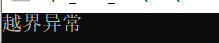运算符
v1 == v2  //比较形式和string一样
v1 != v2
v1 <= v2
v1 >= v2
v1 < v2
v1 > v2
v[]

assign() 给vector重新赋值，之前的元素全部清除，在赋值
	vector<int> myVector(5, 10);//5个元素，每个元素为10
vector<int>::iterator ite = myVector.begin();
for (ite; ite != myVector.end(); ite++)
{
cout << *ite << '\t';
}
cout << "\n";

myVector.assign(3, 2);//重新赋值为3个元素，每个元素为2
ite = myVector.begin();
for (ite; ite != myVector.end(); ite++)
{
cout << *ite << '\t';
}
cout << "\n";

myVector.assign(8, 0);//重新赋值为6个元素，每个元素为0
ite = myVector.begin();
for (ite; ite != myVector.end(); ite++)
{
cout << *ite << '\t';
}
cout << "\n";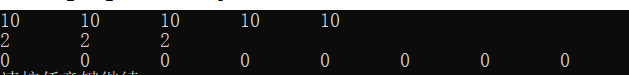front()返回vector起始元素
back()返回vector的最后一个元素
	vector<int> myVector(5, 0);//5个元素，每个元素为0
//给myVector每个下标赋值
for (int i = 0; i < 5; i++)
{
myVector.at(i) = i;
}

//遍历vector
vector<int>::iterator ite = myVector.begin();
for (ite; ite != myVector.end(); ite++)
{
cout << *ite << '\t';
}
cout << "\n";

cout << "起始元素: " << myVector.front() << "\n";
cout << "最后一个元素: " << myVector.back() << "\n";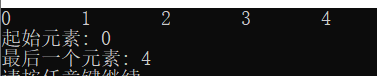push_back()，在末尾添加元素
pop_back()，尾删除
注：没有头添加和头删除
	vector<int> myVector(5, 0);//5个元素，每个元素为0
//给myVector每个下标赋值
for (int i = 0; i < 5; i++)
{
myVector.at(i) = i;
}

//遍历vector
vector<int>::iterator ite = myVector.begin();
for (ite; ite != myVector.end(); ite++)
{
cout << *ite << '\t';
}
cout << "\n";

//尾添加
myVector.push_back(100);
myVector.push_back(200);

ite = myVector.begin();
for (ite; ite != myVector.end(); ite++)
{
cout << *ite << '\t';
}
cout << "\n";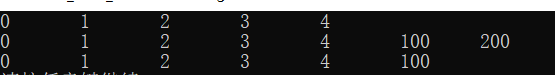capacity()返回容量大小
也就是返回vector所能容纳的元素数量(在不重新分配内存的情况下）
容量不够，每次容量增加原容量的一半
	vector<int> myVector(5, 0);//5个元素，每个元素为0
//给myVector每个下标赋值
for (int i = 0; i < 5; i++)
{
myVector.at(i) = i;
}

//遍历vector
vector<int>::iterator ite = myVector.begin();
for (ite; ite != myVector.end(); ite++)
{
cout << *ite << '\t';
}
cout << "\n";

cout << myVector.capacity() << "\n";//5
myVector.push_back(10);
//容量不够，每次容量增加原容量的一半 5 + 5/2 = 7
cout << myVector.capacity() << "\n";//7
myVector.push_back(10);
myVector.push_back(10);
cout << myVector.capacity() << "\n";//10
myVector.push_back(10);
myVector.push_back(10);
myVector.push_back(10);
cout << myVector.capacity() << "\n";//15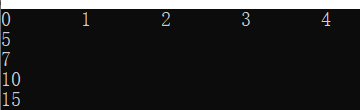reserve()改变容量大小，只能变大，不能变小
设置变大后，容量就为设置的大小
设置变小后，容量还是之前的大小，不改变
	vector<int> myVector(5, 0);//5个元素，每个元素为0
//给myVector每个下标赋值
for (int i = 0; i < 5; i++)
{
myVector.at(i) = i;
}

//遍历vector
vector<int>::iterator ite = myVector.begin();
for (ite; ite != myVector.end(); ite++)
{
cout << *ite << '\t';
}
cout << "\n";

cout << "容量大小：" << myVector.capacity() << "\n";//5
//cout << "元素个数：" << myVector.size() << "\n";//5

myVector.reserve(18);
cout << "reserve(18)后" << "\n";
cout << "容量大小：" << myVector.capacity() << "\n";//18

myVector.reserve(5);
cout << "reserve(5)后" << "\n";
cout << "容量大小：" << myVector.capacity() << "\n";//18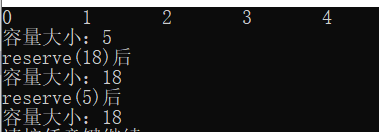resize()使用，改变vector的元素个数
原型：
void resize (size_type n, value_type val = value_type());
第一个参数是修改元素的个数，
第二个参数，
如果修改的元素个数大于之前的元素个数，第二个参数才起作用，扩充的元素位置都为该元素，如果不写该参数，那么默认为0
如果修改的元素个数小于之前的元素个数，第二个参数不起作用
注：如果修改的元素个数大于之前的元素个数，容量大小也会变化为修改后的元素个数
	vector<int> myVector(5, 0);//5个元素，每个元素为0
//给myVector每个下标赋值
for (int i = 0; i < 5; i++)
{
myVector.at(i) = i;
}

//遍历vector
vector<int>::iterator ite = myVector.begin();
for (ite; ite != myVector.end(); ite++)
{
cout << *ite << '\t';
}
cout << "\n";

cout << "容量大小：" << myVector.capacity() << "\n";//5
cout << "元素个数：" << myVector.size() << "\n";//5

cout << "====resize(3)元素个数变小后====" << "\n";
myVector.resize(3);//改变vector的大小并重新赋值
cout << "容量大小：" << myVector.capacity() << "\n";//7
cout << "元素个数：" << myVector.size() << "\n";//3

//遍历vector
ite = myVector.begin();
for (ite; ite != myVector.end(); ite++)
{
cout << *ite << '\t';
}
cout << "\n";

cout << "====resize(8)元素个数变大后====" << "\n";
myVector.resize(8);//改变vector的大小并重新赋值
//这里容量大小，是跟随resize的大小变化
cout << "容量大小：" << myVector.capacity() << "\n";//15
cout << "元素个数：" << myVector.size() << "\n";//11

//遍历vector
ite = myVector.begin();
for (ite; ite != myVector.end(); ite++)
{
cout << *ite << '\t';
}
cout << "\n";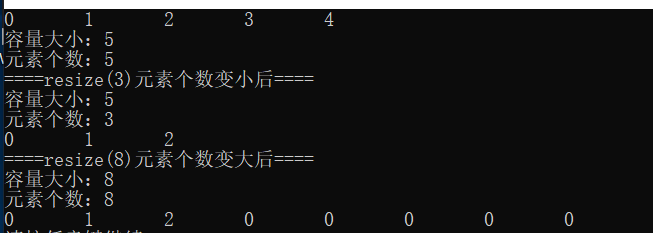上述代码，改变resize(8, 99);
结果如下：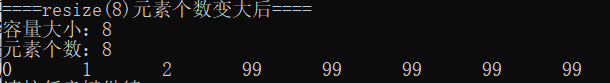empty()，判断元素是否为空
clear()，清空元素
	vector<int> myVector(5, 0);//5个元素，每个元素为0
//给myVector每个下标赋值
for (int i = 0; i < 5; i++)
{
myVector.at(i) = i;
}

//遍历vector
vector<int>::iterator ite = myVector.begin();
for (ite; ite != myVector.end(); ite++)
{
cout << *ite << '\t';
}
cout << "\n";

//判断元素是否为空
cout << boolalpha << myVector.empty() << "\n";

//清空元素
myVector.clear();

cout << boolalpha << myVector.empty() << "\n";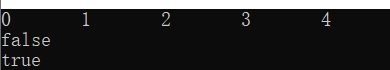insert插入
	vector<int> myVector;//5个元素，每个元素为0

myVector.push_back(4);
myVector.push_back(5);
myVector.push_back(6);

//遍历vector
vector<int>::iterator ite = myVector.begin();
for (ite; ite != myVector.end(); ite++)
{
cout << *ite << '\t';
}
cout << "\n\n";

cout << "insert(ite, 3);后" << "\n";
ite = myVector.begin();
myVector.insert(ite, 3);//插入元素3

//遍历vector
ite = myVector.begin();
for (ite; ite != myVector.end(); ite++)
{
cout << *ite << '\t';
}
cout << "\n\n";

cout << "insert(myVector.end(), 2, 0);后" << "\n";
myVector.insert(myVector.end(), 2, 0);//插入2个66元素

//遍历vector
ite = myVector.begin();
for (ite; ite != myVector.end(); ite++)
{
cout << *ite << '\t';
}
cout << "\n\n";

cout << "insert(myVector.begin(), vectorInsert.begin(), vectorInsert.end());后" << "\n";
vector<int> vectorInsert;
vectorInsert.push_back(0);
vectorInsert.push_back(1);
vectorInsert.push_back(2);

myVector.insert(myVector.begin(), vectorInsert.begin(), vectorInsert.end());

//遍历vector
ite = myVector.begin();
for (ite; ite != myVector.end(); ite++)
{
cout << *ite << '\t';
}
cout << "\n\n";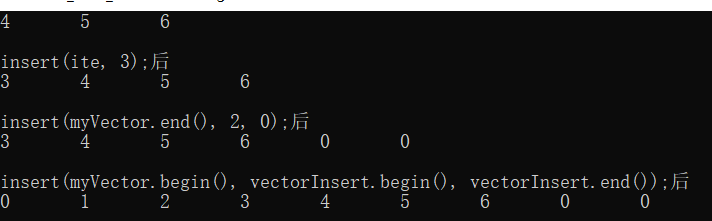erase() 删除指定位置或某个区间（前闭后开）的元素
	vector<int> myVector;//5个元素，每个元素为0

myVector.push_back(4);
myVector.push_back(5);
myVector.push_back(6);
myVector.push_back(7);
myVector.push_back(8);

//遍历vector
vector<int>::iterator ite = myVector.begin();
for (ite; ite != myVector.end(); ite++)
{
cout << *ite << '\t';
}
cout << "\n\n";

ite = myVector.end();
ite--;
myVector.erase(ite);//删除ite位置的元素

ite = myVector.begin();
for (ite; ite != myVector.end(); ite++)
{
cout << *ite << '\t';
}
cout << "\n\n";

ite = myVector.end();
ite -= 2;
//返回值是指向删除的最后一个元素的下一个位置的迭代器
vector<int>::iterator tmp = myVector.erase(myVector.begin(), ite);//删除指定区间的元素

ite = myVector.begin();
for (ite; ite != myVector.end(); ite++)
{
cout << *ite << '\t';
}
cout << "\n\n";

cout << "查看删除元素后的vector的首元素为：" << *tmp << "\n";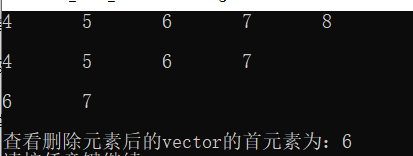swap()交换两个vector元素
	vector<int> myVector;//5个元素，每个元素为0

myVector.push_back(4);
myVector.push_back(5);
myVector.push_back(6);

vector<int> myVector2(2, 0);

//遍历vector
vector<int>::iterator ite = myVector.begin();
for (ite; ite != myVector.end(); ite++)
{
cout << *ite << '\t';
}
cout << "\n\n";

//遍历vector
ite = myVector2.begin();
for (ite; ite != myVector2.end(); ite++)
{
cout << *ite << '\t';
}
cout << "\n\n";

//交换两个vector
myVector.swap(myVector2);

//遍历vector
ite = myVector.begin();
for (ite; ite != myVector.end(); ite++)
{
cout << *ite << '\t';
}
cout << "\n\n";

//遍历vector
ite = myVector2.begin();
for (ite; ite != myVector2.end(); ite++)
{
cout << *ite << '\t';
}
cout << "\n\n";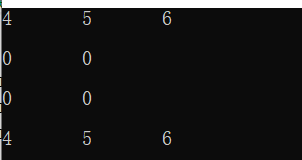展开全文• ## Vector

2019-08-07 17:26:46
vector简介 vector是序列式容器(sequence containers)中的一种。 向量（vector）是一个封装了动态大小数组的顺序容器（Sequence Container）。跟任意其它类型容器一样，它能够存放各种类型的对象。可以简单的认为...
• １，简述一下vector的基本操作，它的ｓｉｚｅ，capacity（），ｃｌｅａｒ，ｒｅｖｅｒｓｅ，ｒｅｓｅｒｖｅ， 　push_back等！！！ ２，说说，ｖｅｃｔｏｒ的存储特性，是顺序存储还是如同链表般，如果是顺序存储...
•vector
• 文章目录一、头文件 一、头文件 在std中，所以只用打 using namespace std;
• C++ vector的用法（整理） vector 是向量类型，它可以容纳许多类型的数据，如若干个整数，所以称其为容器。vector 是C++ STL的一个重要成员，使用它时需要包含头文件： #include&lt;vector&gt;; 一、...
• 介绍这篇文章的目的是为了介绍std::vector，如何恰当地使用它们的成员函数等操作。本文中还讨论了条件函数和函数指针在迭代算法中使用，如在remove_if()和for_each()中的使用。通过阅读这篇文章读者应该能够有效地...vector iterator 数据结构 算法 c
• (1): vector&lt;int&gt; ilist1; 默认初始化，vector为空， size为0，表明容器中没有元素，而且 capacity 也返回 0，意味着还没有分配内存空间。这种初始化方式适用于元素个数未知，需要在程序中动态添加的...
• 1、为什么需要主动释放vector内存 vector其中一个特点：内存空间只会增长，不会减小，援引C++ Primer：为了支持快速的随机访问，vector容器的元素以连续方式存放，每一个元素都紧挨着前一个元素存储。设想一下，当...vector swap empty
•  向量 vector 是一种对象实体, 能够容纳许多其他类型相同的元素, 因此又被称为容器。 与string相同, vector 同属于STL(Standard Template Library, 标准模板库)中的一种自定义的数据类型, 可以广义上认为是数组的...
• 简介 在STL中Vector这一容器，无论是在封装程度还是内存管理等方面都由于传统C++中的数组。...（1）利用Vector的push_back函数vector<vector<int>> vec;vector<int> a; a.push_back(1); a.push_back(2); a.push_bvector 二维数组
• 今天写代码的是遇到想对vector进行排序的问题，隐约记得std::sort函数是可以对vector进行排序的，但是这次需要排序的vector中压的是自己定义的结构体（元素大于等于2），想以其中某一个元素进行正序或逆序排序，则不...vector sort 排序 结构体
• vector 是C++ STL的一个重要成员，使用它时需要包含头文件： #include<vector>; 一、vector 的初始化：可以有五种方式,举例说明如下： (1) vector<int> a(10); //定义了10个整型元素的向量（尖...vector
• 前天要做一个对C++ STL的vector容器做一个排序操作，之前一直把vector当做一个容量可自动变化的数组，是的，数组，所以打算按照对数组进行排序的方法：用快速排序或是冒泡排序等算法自己写一个排序的函数。...vector iterator struct
• 今天又忘了怎么在vector中查找某一个值。。唉。。每次都忘。。现在记下来。。（- -!） stl包括容器、迭代器和算法： 容器 用于管理一些相关的数据类型。每种容器都有它的优缺点，不同的容器反映出...vector 算法 iterator algorithm 编程
• 标准库vector类型是C++中使用较多的一种类模板，vector类型相当于一种动态的容器，在vector中主要有一些基本的操作，接下来分别从以下的几个方面总结： vector对象的定义和初始化 vector对象的基本操作，主要包括...vector C
...